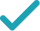Type a math problemSolve for xSolution Steps
Variable cannot be equal to since division by zero is not defined. Multiply both sides of the equation by .
To multiply powers of the same base, add their exponents. Add and to get .
Rearrange the equation to put it in standard form. Place the terms in order from highest to lowest power.
By Rational Root Theorem, all rational roots of a polynomial are in the form , where divides the constant term and divides the leading coefficient . List all candidates .
Find one such root by trying out all the integer values, starting from the smallest by absolute value. If no integer roots are found, try out fractions.
By Factor theorem, is a factor of the polynomial for each root . Divide by to get . Solve the equation where the result equals to .
All equations of the form can be solved using the quadratic formula: . Substitute for , for , and for in the quadratic formula.
Do the calculations.
Solutions are the same.
List all found solutions.
GraphGraph Both Sides in 2D
Graph in 2DGiving is as easy as 1, 2, 3
Get 1,000 points to donate to a school of your choice when you join Give With Bing
x^{2}x+x^{2}\left(-3\right)+4=0
Variable x cannot be equal to 0 since division by zero is not defined. Multiply both sides of the equation by x^{2}.
x^{3}+x^{2}\left(-3\right)+4=0
To multiply powers of the same base, add their exponents. Add 2 and 1 to get 3.
x^{3}-3x^{2}+4=0
Rearrange the equation to put it in standard form. Place the terms in order from highest to lowest power.
±4,±2,±1
By Rational Root Theorem, all rational roots of a polynomial are in the form \frac{p}{q}, where p divides the constant term 4 and q divides the leading coefficient 1. List all candidates \frac{p}{q}.
x=-1
Find one such root by trying out all the integer values, starting from the smallest by absolute value. If no integer roots are found, try out fractions.
x^{2}-4x+4=0
By Factor theorem, x-k is a factor of the polynomial for each root k. Divide x^{3}-3x^{2}+4 by x+1 to get x^{2}-4x+4. Solve the equation where the result equals to 0.
x=\frac{-\left(-4\right)±\sqrt{\left(-4\right)^{2}-4\times 1\times 4}}{2}
All equations of the form ax^{2}+bx+c=0 can be solved using the quadratic formula: \frac{-b±\sqrt{b^{2}-4ac}}{2a}. Substitute 1 for a, -4 for b, and 4 for c in the quadratic formula.
x=\frac{4±0}{2}
Do the calculations.
x=2
Solutions are the same.
x=-1 x=2
List all found solutions.